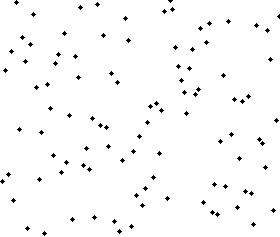0
0
01. 云栖社区>
2. 博客>
3. 正文

冒泡排序

2 7 4 6 9 1 首先比较最后两个数字，发现1比9小，于是前移

2 7 4 6 1 9 然后比较6和1

2 7 4 1 6 9 继续前移，然后是4和1

7 1 4 6 9 7和1比较

2 1 7 4 6 9 2和1

1 2 7 4 6 9 至此，第一趟冒泡过程完成，最小的元素1被移到第一个，不再参与后面的排序过程。下一趟冒泡过程同理，比较6和9，以此类推，最终得到结果。

 1 2 3 4 5 6 7 8 9 10 11 12 void bubbleSort(int arr[],int n) {     int i,j,t;      for(i=0;i

测试代码如下：

1．比较相邻的前后二个数据，如果前面数据大于后面的数据，就将二个数据交换。

2．这样对数组的第0个数据到N-1个数据进行一次遍历后，最大的一个数据就“沉”到数组第N-1个位置。

3．N=N-1，如果N不为0就重复前面二步，否则排序完成。

 1 2 3 4 5 6 7 8 9 //冒泡排序1 void BubbleSort1(int a[], int n) {        int i, j;        for (i = 0; i < n; i++)               for (j = 1; j < n - i; j++)                      if (a[j - 1] > a[j])                             Swap(a[j - 1], a[j]); }

下面对其进行优化，设置一个标志，如果这一趟发生了交换，则为true，否则为false。明显如果有一趟没有发生交换，说明排序已经完成。

 1 2 3 4 5 6 7 8 9 10 11 12 13 14 15 16 17 18 19 20 //冒泡排序2 void BubbleSort2(int a[], int n) {        int j, k;        bool flag;          k = n;        flag = true;        while (flag)        {               flag = false;               for (j = 1; j < k; j++)                      if (a[j - 1] > a[j])                      {                             Swap(a[j - 1], a[j]);                             flag = true;                      }               k--;        } }

再做进一步的优化。如果有100个数的数组，仅前面10个无序，后面90个都已排好序且都大于前面10个数字，那么在第一趟遍历后，最后发生交换的位置必定小于10，且这个位置之后的数据必定已经有序了，记录下这位置，第二次只要从数组头部遍历到这个位置就可以了。

 1 2 3 4 5 6 7 8 9 10 11 12 13 14 15 16 17 18 19 //冒泡排序3 void BubbleSort3(int a[], int n) {     int j, k;     int flag;           flag = n;     while (flag > 0)     {         k = flag;         flag = 0;         for (j = 1; j < k; j++)             if (a[j - 1] > a[j])             {                 Swap(a[j - 1], a[j]);                 flag = j;             }     } }

冒泡排序毕竟是一种效率低下的排序方法，在数据规模很小时，可以采用。数据规模比较大时，最好用其它排序方法。

int bubble_sort(vector<int> &v){
cout << "bubble sort:" << endl;
printline("before sort:", v);
bool exchange;
for (int i=0; i<v.size(); i++){
int temp = 0;
exchange = false;
for(int j=v.size()-1; j>0; j--){
if (v[j] < v[j-1]){
temp = v[j];
v[j] = v[j-1];
v[j-1] = temp;
exchange = true;
}
}
if (!exchange){
break;
}
}
printline("after sort:",v);
}+ 关注